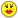+0

# if f(x/((x^2)+x+1)=(x^2)/((x^4)+(x^2)+1) , then f(2)=?

0
1082
8

if f(x/((x^2)+x+1)=(x^2)/((x^4)+(x^2)+1) , then f(2)=?

Apr 14, 2015

#7
+5

Thanks Alan but Now what?

-------------------------------------------

You are suppose to eat the virtual cake, not just look at it.

It is not just for decoration you know :)

Apr 17, 2015

#1
0

$$\\f(x/((x^2)+x+1)=(x^2)/((x^4)+(x^2)+1) \\\\ f\left(\frac{x}{(x^2)+x+1}\right)=\frac{(x^2)}{(x^4)+(x^2)+1}\qquad find\;f(2)\\\\\\$$

Are you sure you copied the question correctly ??????

Apr 15, 2015
#2
0

yes, it's exactly what you wrote and i found the answer. f(2) = -4/3

in this question, you should imagine that x+(1/x) = -1/2 although this is not possible.

Thanks.

Apr 16, 2015
#3
+5

PLEASE CAN WE GET SOME HELP HERE$$\\f(x/((x^2)+x+1)=(x^2)/((x^4)+(x^2)+1) \\\\ f\left(\frac{x}{(x^2)+x+1}\right)=\frac{(x^2)}{(x^4)+(x^2)+1}\qquad find\;f(2)\\\\\\$$

Now I divided

$$\\(x^4+x^2+1) \;\;by\;\;(x^2+x+1) \;\;and\;\;got\;\;(x^2-x+1)\\\\ so\\\\ (x^2+x+1)\times (x^2-x+1)=(x^4+x^2+1)\\\\ so\\\\ \frac{x}{(x^2+x+1)}\times \frac{x}{(x^2-x+1)}=\frac{x^2}{(x^4+x^2+1)}\\\\ so\\\\ Now I am confused again\\\\$$Apr 17, 2015
#4
+5

Here are my thoughts:The values of the function are complex in the approximate range 0.17<X<5.83.

Outside this range they are real.

.

Apr 17, 2015
#5
0

Thanks Alan,

That makes me feel better, it definitely is not simple!

Perhaps it was meant to be a perfect square so that it would fall into place easily.

BUT what was the  x+1/x = -1/2     for ?

---------------------

Make sure you get some of my cake Alan, there is plenty.  Here is your peice :)

EnjoyApr 17, 2015
#6
+5

If you take

$$\frac{x}{x^2+x+1}=2$$

turn this upside down:

$$\frac{x^2+x+1}{x}=\frac{1}{2}$$

Simplify:

$$x+1+\frac{1}{x}= \frac{1}{2}$$

Rearrange:

$$x+\frac{1}{x}=-\frac{1}{2}$$

The picture of the cake is making my mouth water!

.

Apr 17, 2015
#7
+5

Thanks Alan but Now what?

-------------------------------------------

You are suppose to eat the virtual cake, not just look at it.

It is not just for decoration you know :)

Melody Apr 17, 2015
#8
0

I feel a bit of a fraud coming in on this one after the introductory work done by the three of you, providing the ideas and so on, however,

We need

$$\displaystyle \frac{x}{x^{2}+x+1}\quad \text{to equal} \quad 2.$$

Turn that upside down, as suggested by Chris, and we have

$$\displaystyle x+1+\frac{1}{x}=\frac{1}{2}\quad \dots(1).$$

Now look at the rhs, suppose that's equal to $$k$$.

Turn that upside down and we have

$$\displaystyle x^{2}+1+\frac{1}{x^{2}}=\frac{1}{k}.$$

Squaring (1),

$$\displaystyle x^{2}+1+\frac{1}{x^{2}}+2x+2+\frac{2}{x}=\frac{1}{4},$$

so

$$\displaystyle x^{2}+1+\frac{1}{x^{2}}= \frac{1}{4}-2\left(x+1+\frac{1}{x}\right)=\frac{1}{4}-\left(2\times\frac{1}{2}\right)=-\frac{3}{4},$$

in which case

$$\displaystyle \frac{1}{k}=-\frac{3}{4},\quad \text{so} \qquad k=-\frac{4}{3}.$$

Apr 17, 2015